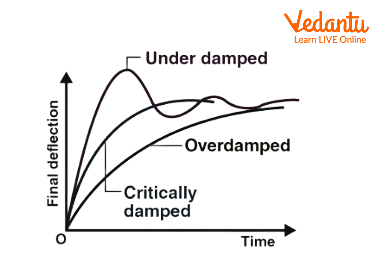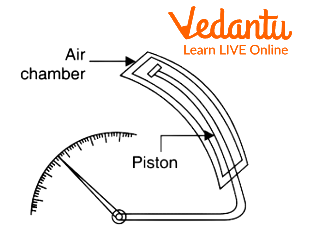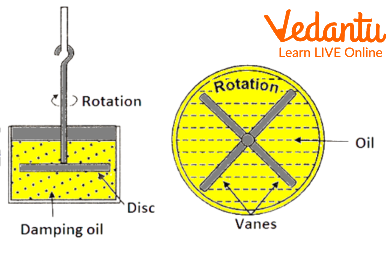Courses
Courses for Kids
Free study material
Offline Centres
More

# Damping TorqueLast updated date: 05th Dec 2023
Total views: 115.2k
Views today: 1.15k## Learning the Importance of Damping Torque

For an instrument to indicate the readings, it needs to have three important systems called a deflection system, controlling system and damping system, which respectively, provide three torques called as deflecting torque, controlling torque and damping torque. These three torques are necessary for indicating instruments.

The use of damping torque is to remove oscillation and vibration and bring the pointer at rest in a quick manner in indicating instruments. In other words, we can say that damping torque is required to stop the vibration of the pointer to read accurate value on the scale.

## Importance of Damping Torque

Damping torque is the torque which is required to reduce the number of oscillations. If the damping torque is absent, it will require several time-consuming processes in order to take each and every reading. Here lies the importance of damping torque in all indicating type analogue instruments.

## Main Function of Damping Torque

The main function of damping torque is to damp or reduce the number of oscillations that the pointer or the deflecting system undergoes. As the number of oscillations is reduced, it comes to steady or equilibrium state faster and the reading can be taken quickly. We can represent three conditions of this damping torque by a graph shown here.Dynamic Response of a Measuring Instrument

The above graph shows the conditions of damping torque. The behaviour of the moving system can be decided from the degree of damping.

• From the above graph, we can say that the pointer will become slow, if the instrument is over-damped.

• Critical damping is when the pointer returns to its equilibrium position in a small amount of time without doing any oscillation. Instruments such as speedometers come under this category.

• When the response is oscillatory, we can say that the instrument is under-damped.

## Requirement of Damping Torque

To bring the moving system to a stop, damping torque is always required in the measuring instrument and it exhibits stable reflection in an acceptable amount of time. In other words, we can say that to bring the moving system to rest to indicate steady reflection in a very short amount of time, damping torque is required.

## Different Types of Methods to Produce Damping Torque

The damping torque can be produced by the following three methods:

• Air Friction Damping

• Fluid Friction Damping

• Eddy Current Damping

In air friction damping torque, a light aluminium vane is attached to the moving system, which moves in an air chamber. The air pressure inside the chamber restricts the movement of the vane and provides required damping torque.Air Friction Damping

Air friction damping method is very simple and low maintenance. It does not require a permanent magnet and hence there would be no problem of distortion.

• Generally, air friction damping is used where low value of the magnetic field is required.

• Air friction damping is generally used in moving iron and electrodynamometer type instruments. Moving iron type instruments can work on both AC and DC.

• Air friction damping is used in thermal instruments as well.

In fluid friction damping, a light aluminium vane is attached to the moving system, which is placed inside the fluid container so that friction offered by the fluid can oppose the motion of the pointer. The friction offered by liquid is more compared to the friction offered by air. So, fluid friction can provide more damping force.

• Fluid friction damping is generally used for laboratory type electrostatic type instruments.

• It is used in wall mounted type instruments as well.Fluid Friction Damping

Due to leakage of fluid, it is not easy to keep the instrument clean every time. Moreover, it requires more maintenance and its cost is also very high. Fluid viscosity should not change with temperature. It is not suitable for repeated operations. These are the major drawbacks of fluid friction damping. Apart from that, fluid should not evaporate quickly. Also, fluid should not have any corrosive action upon the metal of the instrument. So, these are the drawbacks of the fluid friction damping method.

## Eddy Current Damping

According to Faraday’s law, whenever the change takes place in the magnetic flux which is linked with the circuit, an emf current is induced in the circuit. This current can produce an electromagnetic torque due to the magnetic field. This will oppose the direction of motion. Hence, electromagnetic torque provides the necessary damping force.

• Eddy current damping is used where a high value of the magnetic field is required. For instance, eddy current damping is generally used in Permanent Magnetic Moving Coil which is known as PMMC and it is an instrument which is used to measure current or voltage. The advantage of PMMC is that it has less power consumption and high accuracy.

• Eddy current damping is also used in induction type instruments.

## Solved Examples

1. A PMMC ammeter has a rectangular coil $2cm \times 3cm$ with 100 turns and is placed in a uniform magnetic field of density $0.1\dfrac{{Wb}}{{{m^2}}}$ The spring constant is $2.0 \times {10^{ - 7}}$ Nm per degree. Find the deflection of the coil, when it carries a current of 10mA ac.

Ans: Given: $N = 100turns$

spring constant $k = 2.0 \times {10^{ - 7}}$ Nm per degree

Magnetic field $B = 0.1\dfrac{{Wb}}{{{m^2}}}$

The deflection of the coil will be zero in case of alternating current. This means that there will be no deflection, since average torque is zero in case of an ac.

2. The torque of an ammeter varies as the square of the current through it. If a current of 10A produces a deflection of $90^\circ$, what deflection will occur for a current of 5A when the instrument is spring controlled?

Ans: Given:

$\begin{array}{l}{I_1} = 10A\\{\theta _1} = 90^\circ \\{I_2} = 5A\end{array}$

In spring control, deflection is proportional to $\theta$

So, we can say that current is proportional to $\theta$

$\begin{array}{l}\therefore \dfrac{{{I_1}}}{{{I_2}}} = \dfrac{{{\theta _1}}}{{{\theta _2}}}\\ \Rightarrow \dfrac{{10}}{5} = \dfrac{{90^\circ }}{{{\theta _2}}}\end{array}$

$\therefore {\theta _2} = \dfrac{{5 \times 90^\circ }}{{10}} = 45^\circ$

## Interesting Facts

• For the electro dynamometer type instruments, we can not use eddy current damping because the operating field is very weak.

• Damping is necessary for an electromechanical measuring instrument.

• Moving coil galvanometer is not suitable to measure alternating current.

## Conclusion

The damping torque in indicating type instrument can be provided by air friction damping, fluid friction damping and eddy current damping method. Eddy current damping is the most efficient method as compared to the other methods. This was the complete discussion on damping torque and its importance. We hope you have found it interesting and informative enough. Try to solve the above questions on your own and visit our website for further reading on similar topics.

## FAQs on Damping Torque

1. State different types of torque which can be used for measuring instruments and also explain the importance of controlling torque.

There are three different types of torque which can be used for measuring instruments. They are deflecting torque, controlling torque and damping torque.

Controlling torque plays an important role in measuring instruments. Controlling torque opposes the deflecting torque and it increases with the moving system’s deflection. This torque provides us the value of the deflection which generally depends upon the magnitude of the quantity being measured. When this torque becomes equal to the deflecting torque, the pointer will attain steady position. This is how we can understand the importance of controlling torque in measuring instruments.

2. What do we mean by damping?

In our nature, everything tends to stay in equilibrium. Damping comes into picture when we try to disturb this equilibrium. In simple words, we can say that it is the decrease in amplitude of an oscillating system. The oscillation that undergoes damping is known as damped oscillation. Frequency of the system does not change during damping.

There are two types of damping: internal damping and external damping. In an internal damping, an oscillating system loses energy due to the extension and compression of the molecules in the system. An external damping is caused by frictional force or air resistance that acts on it. The oscillations of a system in the presence of a resistance force are generally known as damped oscillation.

3. What are the advantages and disadvantages of a PMMC (Permanent Magnet Moving Coil) instrument?

Low power consumption that means very small power is required for its operation, high torque, very effective eddy current damping can be used without any extra damping mechanism, no hysteresis loss. These are the advantages of the PMMC (Permanent Magnet Moving Coil) instrument. This PMMC instrument can only be used for dc current and voltage measurement. In this instrument, its construction is costly as permanent magnets are used in this instrument. Permanent Magnet Moving Coil instruments are used only for direct current measurements. They can not be used for ac measurements. Strength of the permanent magnet reduces with time and hence error occurs.